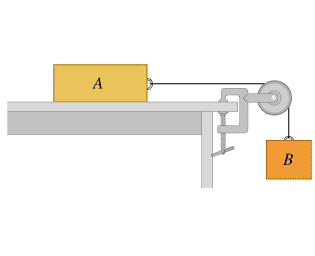# Applying Newton's laws

## Homework Statement

Consider the system shown in the figure (Figure 1) . Block A weighs 46.1 N and block B weighs 28.7 N . Once block B is set into downward motion, it descends at a constant speed.

http://session.masteringphysics.com/problemAsset/1038576/6/YF-05-54.jpgA

Calculate the coefficient of kinetic friction between block A and the tabletop
μ= 0.623

B
A cat, also of weight 46.1 N , falls asleep on top of block A. If block B is now set into downward motion, what is its acceleration magnitude

F=ma

## The Attempt at a Solution

∑F in x direction of A: -F+T = ma
-μ92.1+T = 92.2/ 9.8 a
-.623(92.1)+T=9.4 a

∑F in y of A N-w = ma
92.2-9.4(9.8) = 0

∑F in Y of B T-w
T-2.93 (9.8) = 2.93 a
T= 28.7+2.93a

plug T into first equation: -.623(92.1)+28.7+2.93a = 9.4a
-.623(92.1)+28.7= (9.4-2.93) a
divide both sides
( -.623(92.1)+28.7) /9.4-2.93 = a

gives me wrong answer, where did I go wrong?

I think that the easiest way to solve is to do a free body diagram including the forces of friction, gravity, and tension then proceed to fill in the known values for the forces which should lead to tension canceling out algebraically then you can solve for acceleration

Remember that for each side of the pulley the sum of the forces should equal the mass of the block times the acceleration

PhanthomJay
Homework Helper
Gold Member
What is the direction of the acceleration of each block?

•David112234
Consider the system shown in the figure (Figure 1) . Block A weighs 46.1 N and block B weighs 28.7 N . Once block B is set into downward motion, it descends at a constant speed.

I do not understand why you are calculating 'a ' the acceleration of masses as it should be zero as per your problem.

## Homework Statement

Consider the system shown in the figure (Figure 1) . Block A weighs 46.1 N and block B weighs 28.7 N . Once block B is set into downward motion, it descends at a constant speed.

http://session.masteringphysics.com/problemAsset/1038576/6/YF-05-54.jpg
View attachment 97316
A

Calculate the coefficient of kinetic friction between block A and the tabletop
μ= 0.623

B
A cat, also of weight 46.1 N , falls asleep on top of block A. If block B is now set into downward motion, what is its acceleration magnitude

F=ma

## The Attempt at a Solution

∑F in x direction of A: -F+T = ma
-μ92.1+T = 92.2/ 9.8 a
-.623(92.1)+T=9.4 a

∑F in y of A N-w = ma
92.2-9.4(9.8) = 0

∑F in Y of B T-w
T-2.93 (9.8) = 2.93 a
T= 28.7+2.93a

plug T into first equation: -.623(92.1)+28.7+2.93a = 9.4a
-.623(92.1)+28.7= (9.4-2.93) a
divide both sides
( -.623(92.1)+28.7) /9.4-2.93 = a

gives me wrong answer, where did I go wrong?
So i have looked through your original post and i have found some problems. Like -μ"92.1"+T = 92.2/ 9.8 a => "92,2"
But i suggest you using this formula : a = ΣF / ΣM, more faster and i think that you should start again rather than try figuring the mistake

A cat, also of weight 46.1 N , falls asleep on top of block A. If block B is now set into downward motion, what is its acceleration magnitude

## Homework Equations

F=ma

In the above case is it possible that the accelerated motion take place?

In the above case is it possible that the accelerated motion take place?
I think that the friction force is greater than gravity so the system won't move

PhanthomJay
Homework Helper
Gold Member
I do not understand why you are calculating 'a ' the acceleration of masses as it should be zero as per your problem.
it is zero in part a but non zero in part b. The error is in part b.

I think that the easiest way to solve is to do a free body diagram including the forces of friction, gravity, and tension then proceed to fill in the known values for the forces which should lead to tension canceling out algebraically then you can solve for acceleration

Remember that for each side of the pulley the sum of the forces should equal the mass of the block times the acceleration
That is what I did, or at least tried

What is the direction of the acceleration of each block?
A is left
B is up

A is left
B is up

which force is moving the body A towards the left?

So i have looked through your original post and i have found some problems. Like -μ"92.1"+T = 92.2/ 9.8 a => "92,2"
But i suggest you using this formula : a = ΣF / ΣM, more faster and i think that you should start again rather than try figuring the mistake

What is wrong with what I posted?
The friction force u92.2 is pulling left and Tension pulling right
which force is moving the body A towards the left?
Friction

Got it, I was missing a negative sign, thanks

Last edited:
Friction
friction can not move -it can only provide resistance to motion-
if your body is pulled left it will oppose it

again if your body gets pulled towards right it will oppose it - so will there be motion towards right if it opposes the motion?

friction can not move -it can only provide resistance to motion-
if your body is pulled left it will oppose it

again if your body gets pulled towards right it will oppose it - so will there be motion towards right if it opposes the motion?

Yes the motion is to the right but the acceleration is to the left so the object is slowing down due to the friction force which acts towards the left. I figured out the answer, my mistake was not putting a negative sign in front of the acceleration

•drvrm